SBAA534 March   2022

# 1 Component and Topology Selection - Finding a Good Starting Point Quickly

The following chapters use an example design described by Table 1-1.

Table 1-1 Design Goal Parameters of Example Analog Front-End
Parameter Design Goal
Channel Count 8
Bandwidth and Sampling Rate 40 kHz, > 100 ksps
Input Full Scale Range (FSR) ±10-V FSR

Common-Mode Voltage

Target Resolution, ENOB

> 16 bit

Input Impedance (ZIN) Target

> 100 kΩ

TID: > 50 krad, SEL: > 60 (MeV × cm2/mg)

The TI Space Products Guide, Space-Grade Data Converters section shows that the ADS1278-SP best fits these requirements.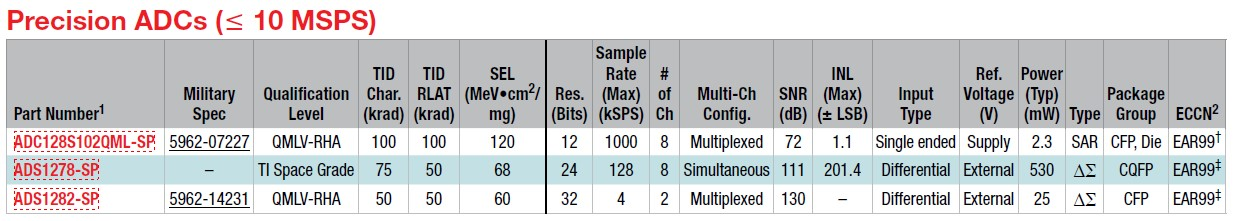Figure 1-1 TI’s Space-Grade Precision ADCs Listed in TI's Space Product Guide

The next step is to identify the right ADC driver. For best noise immunity and linearity a fully differential amplifier (FDA) is preferred.

To identify the right product, the minimum unity gain bandwidth of such FDA must be understood. The ANALOG-ENGINEER’S CALCULATOR provides great assistance here.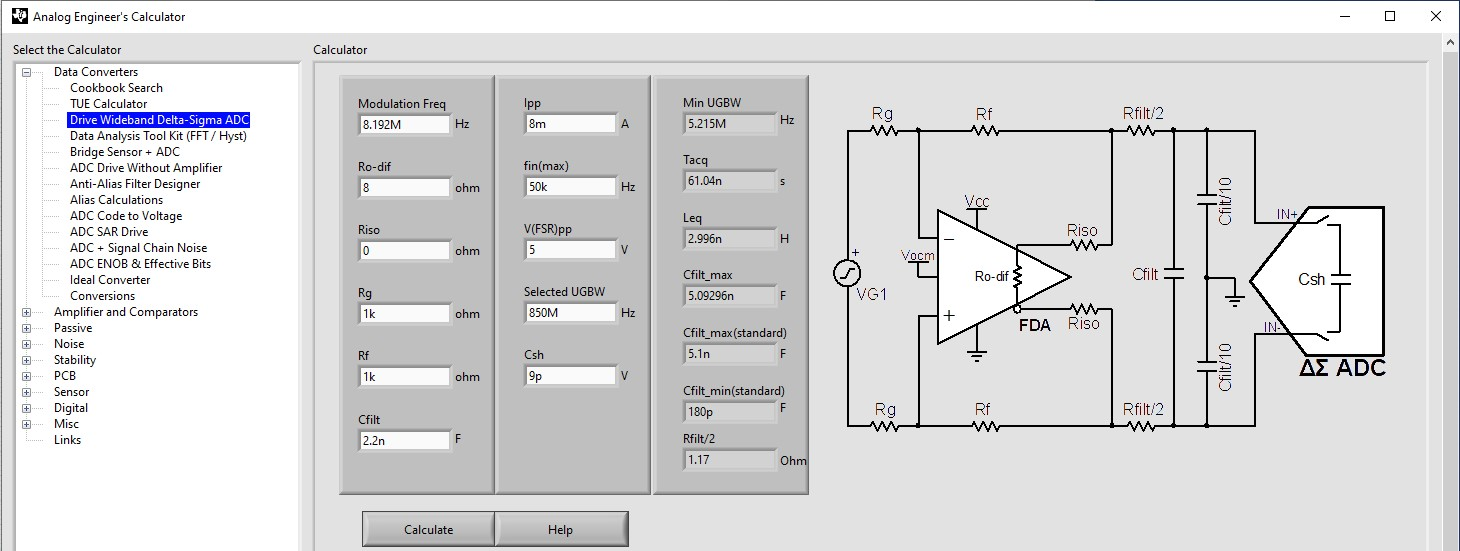Figure 1-2 ANALOG-ENGINEER’S CALCULATOR – Drive Wideband Delta-Sigma ADC

Figure 1-2 illustrates that the Data Converters menu offers the Drive Wideband Delta-Sigma ADC item. The reference schematic in the figure shows all relevant component values. The white fields must be entered by the user, the gray fields are the resulting values. The very first variable to define is the minimum unity gain bandwidth (UGBW). In other words, up to what frequency must the gain of the amplifier be equal or greater than one. Since the ADC will cut off any information above the Nyquist or sample frequency divided by two, it is sufficient if the UGBW of the amplifier is about two-thirds of the sampling frequency. For example, for a sample rate of 1 kHz, look at a minimum UGBW of 636.6 Hz.

The selected ADC in the example of this report is a sigma-delta ADC. It is important to remember that a sigma-delta ADC is a one-bit ADC at the provided modulation frequency. The actual sample rate is only the frequency the full sample words come out of it. The relevant frequency for the AFE design is the modulation frequency which is much higher.

Due to the flexibility and complexity of modern ADCs it is not always straightforward to read the modulation frequency from the data sheet. The ADS1278-SP provides different operating modes that allow for different master clock rates. Figure 1-3 shows the actual modulation frequency is just one-fourth of the master clock. In the example, the ADS1278-SP operates in High-Speed mode: 128 ksps, fCLK is 32.786 MHz. FCLK divided by four results in 8.192 MHz for the actual modulation frequency.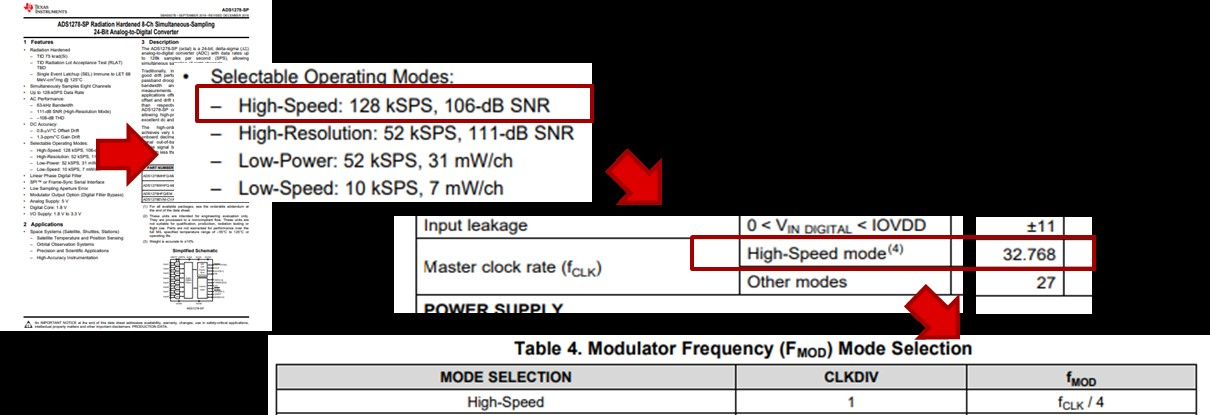Figure 1-3 Reading the Modulation Frequency From the ADS1278-SP Data Sheet
Figure 1-4 shows that entering the modulation frequency of "8.192M" (8.192 MHz) into the ANALOG-ENGINEER’S CALCULATOR determines a minimum UGBW of "5.215M" (5.215 MHz).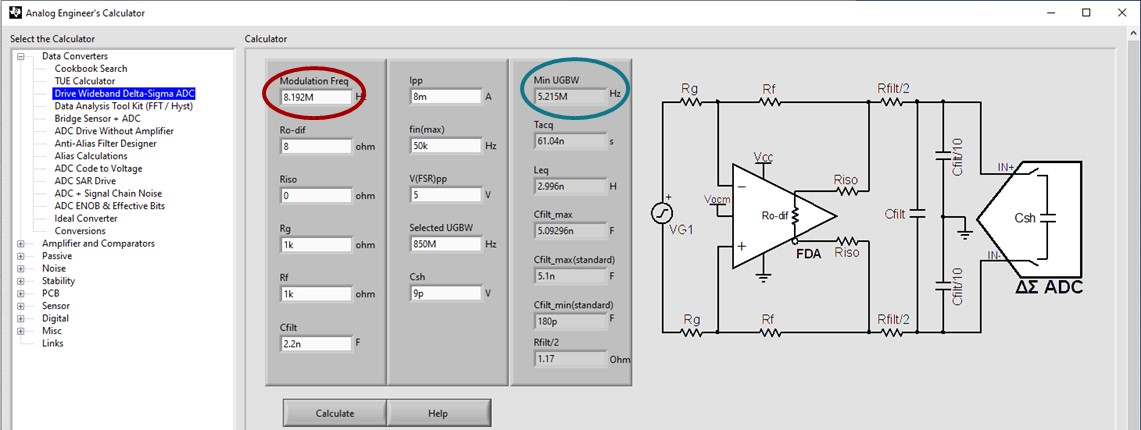Figure 1-4 Using ANALOG-ENGINEER’S CALCULATOR to Determine the Minimum Unity Gain Bandwidth of the FDA

Figure 1-5 illustrates the TI space product guide showing four FDAs. All four provide more than sufficient UGBW. As an example for this report, the lowest one (LMH5485-SP) with 850-MHz UGBW is selected.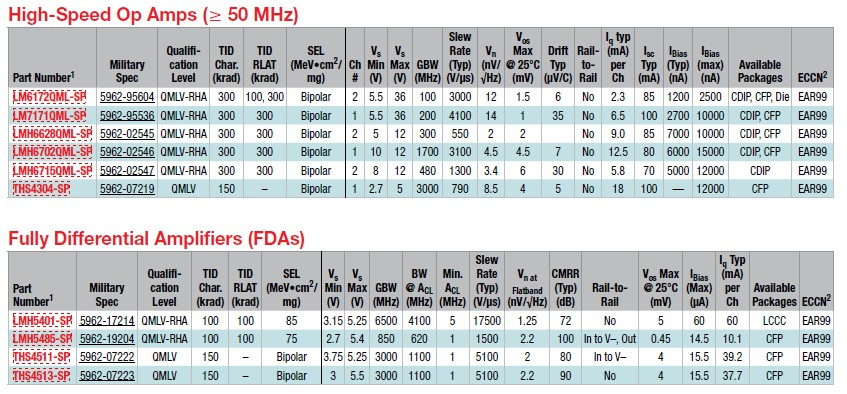Figure 1-5 Selecting the Best Fitting FDA from TI’s Space Product Guide

With that selection it is now possible to fill the rest of the white fields in the ANALOG-ENGINEER’S CALCULATOR, see Figure 1-6.

Parameter Description
Ro-dif Ro-dif is the “open-loop output impedance”. The LMH5485-SP Radiation Hardened Assured (RHA) Negative Rail Input, Rail-to-Rail Output, Precision, 850 MHz Fully Differential Amplifier data sheet provides only the closed-loop output impedance = 0.1 Ω.

The Small-Signal Frequency Response vs Gain graph in the Typical Characteristics: 5 V Single Supply section of the LMH5485-SP data sheet provides the gain versus frequency curve. For the modulation frequency of 8.192 MHz, the graph shows about 38 dB, that is, about 80.

With the relation of
closed-loop output impedance × open-loop gain = open loop output impedance

Ro-dif calculates as follows:

90 × 0.1 Ω = 8 Ω.
Enter "8" (8 Ω) for the Ro-dif field.

Riso Riso is typically tuned to a small value. Here "0" (0 Ω) is used.
Rg and Rf Rg and Rf must be the same for unity gain. "1k" (1 kΩ) is typically a good starting point.
IPP The LMH5485-SP data sheet allows for up to 75 mA of output current. An unnecessarily high output current would cause power loss and heating of the FDA which may cause a drift from the optimal working point. A value of "8m" (8 mA) is enough to make calculations in this example.
fin The required bandwidth is 40 kHz. With some margin, "50k" (50 kHz) is a good selection here.
UGBW The UGBW of FDA is "859M" (850 MHz).
CSH Use CSH from the Equivalent Analog Input Circuitry image in the ADS1278-SP Radiation Hardened 8-Ch Simultaneous-Sampling 24-Bit Analog-to-Digital Converter data sheet: "9p" (9 pF).
CFILT Prior defining leaves CFILT as the last entries - press the Calculate button to obtain the updated values for Cfilt_min "180p" (180 pF) and Cfilt_max "5.1n" (5.1 nF). Use "2.2n" (2.2 nF) as the value somewhat in between 5 nF and 180 pF.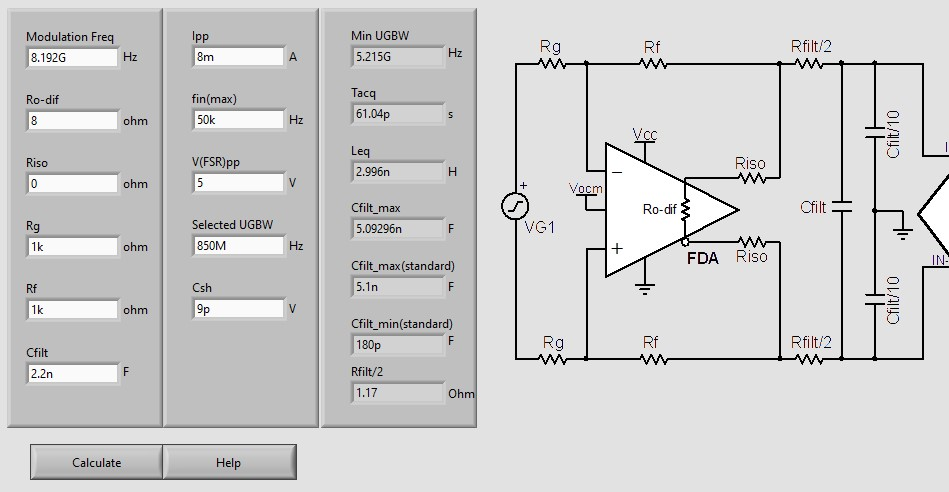Figure 1-6 User Defined And Calculated Values For The Reference Schematic With LMH5485-SP and ADS1278-SP to Meet the Example Design Targets

In this chapter the best fitting ADC and ADC-driver was selected with the help of the TI Space Products Guide and the ANALOG-ENGINEER’S CALCULATOR was used to determine its supporting circuitry, including all component values. Further, a basic understanding of the circuit and the role of each of the active and passive components was developed.